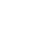# How do we build a "low cost" fast charger for NiCd and NiMH elements?

A cheap charger for charging batteries with elements Ni-Cd or NiMH, easy to build using timer 555 and some transistors. Charging is done quickly, and the electronic scheme ensures the elimination of the unwanted memory effect in NiCd cells.

## What is the memory effect on batteries?

This is as follows: when a NIMH or NICd battery is charged before full discharge, it "stores" the level it had when it was recharged. It becomes "a false threshold", the battery "requesting" then recharging when it reaches the incomplete, "false" discharge threshold, previously reached.

What is interesting in this case is that the use above this false discharge threshold will lead, as you expect, to the extinction of the powered equipment. But, if you force another stop and a start, you will be surprised to see that it still works, which proves that the stop (ie the voltage drop at the terminals) was premature and "false".

In general, trickle charge NiCd batteries, at a current of 1 / 10C for 14h.

However, following the charging curves below, the elements can be charged quickly, following the dV / dt slope or monitoring the battery limit temperature. (38 ° C for NiMH or 35 ° C for NiCd).

## How does the electronic scheme work?

The electronic scheme is designed for 4 elements, model "AA", with effective charging time 1h. A rectangular signal with 3s charge and 10ms discharge is used for charging.

Charging is done at a current of 70mA. SW1 allows the start of the fast charging process of approximately 1 - 1.2h. LED1 signals fast charging in progress, LED2 signals the start of normal charging (at 1 / 10C) when the power supply is connected.

Elements Q7- ZD1 - thermistor TH1 and R14 are optional; they monitor the temperature of the batteries. R14 is chosen for approx. 37 ° C. Q5 is a transistor that supports at least one current 250mA. It can be replaced with BC639 or BD135 for example, in which case it is recommended to mount a resistor in series with the base (4k7… 10k).

#### List of required components (with equivalents)

R1 = 10M
R2 = 470 Ohms;
R3 = 100k;
R4, R5 = 4k7;
R6 = 390 Ohms;
R7 = 100 Ohm / 0.5W;
R8 = 10k;
R9 = 3.3 Ohm / 2.5W
R10 = 33 Ohm / 2.5W
R11 = 1k5;
R12 = 33k;
R13 = 1k;
R14 = is chosen for approx. 37C (for calculation see loading charts);
C1 = 47 uF / 16V
C2 = 470uF;
C3 = 0.1uF;
D1, D2, D3 = 1N4148;
1 x LED1 = green (fast charge indicator);
1 x LED2 = blue (normal charge indicator);
ZD1 = 2.7V zenner diode;
TH1 = thermistor;
Q1 = ZVN4206G (BS170, BS250 or BS108);
Q2, Q3, Q6 = ZTX107 (or BC547, 2N2222);
Q4 = TIP141 (or BDW83C);
Q5 = transistor that supports at least 250mA (BC337, BC817W, 2N3391A etc.);
2 x terminal contacts (for 12V power supply and for output);
1 x socket 4 AA batteries.

Bibliography:

https://www.easylight.ro/
Conex Club Magazine - no.7-8 - 2006
Zetex Handbook Applications

1.betflix say:
2.Edgar R say: